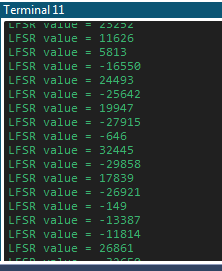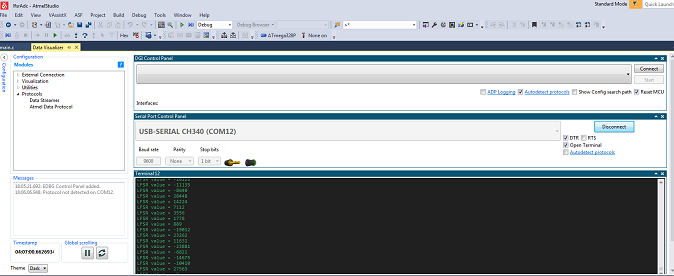# AVR Tutorial – Experiment 4

## Experiment 4

#### Random number generator

Objective – Use LFSR based random number generator to generate a random number and display it.

Pre-Requisites – AVR Motherboard, Mini USB cable, 12V DC Adapter, Software Setup (as described in introduction)

Theory – Random Number generation is a process of obtaining random number within a given range. Using algorithms Pseudo random number generation is possible. There are various methods through which random numbers can be generated on a microcontroller, these are listed as following :-

1. Obtaining the Analog value from a unconnected ADC pin. An unconnected ADC pin may provide a random value due to the noise pickup from surrounding. This method requires the availability of unconnected ADC pin, which may or may not be possible in many cases.
2. Linear Feedback Shift Register (LFSR) – It is based upon taking output from a set of shift registers which is then manipulated to act as the input of the shift register.

The data is sent to the serial monitor on the Atmel Studio IDE using the UART module. The UART module transmits the data which is then interpreted by the serial to USB converter IC (available separately on Arduino Nano), which is then finally transmitted to the computer.

To open terminal go to Tools -> Data Visualizer -> Serial Port Control panel -> select com port -> select baud rate -> connect

Baud rate selected over here is 9600## Connections/Schematic

No connection is required

## Code

``````#define F_CPU 16000000UL
#include <stdlib.h>
#include <avr/io.h>
#include <util/delay.h>

#define LFSR_SEED       (91)

#define BAUDRATE 9600
#define BAUD_PRESCALLER (((F_CPU / (BAUDRATE * 16UL))) - 1)

char buffer;                //Output of the itoa function
uint8_t i=0;                    //Variable for the for() loop

void USART_init(void);            //Function to initialize and configure the USART/serial
void USART_send( unsigned char data);    //Function that sends a char over the serial port
void USART_putstring(char* StringPtr);    //Function that sends a string over the serial port

static uint16_t generate_lfsr_value(void)
{
static uint16_t cnt16 = LFSR_SEED;
return (cnt16 = (cnt16 >> 1) ^ (-(cnt16 & 1) & 0xB400));
}

int main(void)
{
USART_init();        //Setup the USART

/* loop */
while (1) {
uint16_t x= generate_lfsr_value();

USART_putstring("LFSR value = ");
itoa(x, buffer, 10);    //Convert the read value to an ascii string
char *  itoa ( int value, char * str, int base );
USART_putstring(buffer);        //Send the converted value to the terminal
USART_putstring("  ");          //Some more formatting
USART_send('\r');
USART_send('\n');                //This two lines are to tell to the terminal to change line

}
return 0;
}

ADMUX |= (1<<REFS0);                //Voltage reference from Avcc (5v)
ADCSRA |= (1<<ADSC);                //Do an initial conversion because this one is the slowest
//and to ensure that everything is up and running
}

}

void USART_init(void){
UBRR0H = (uint8_t)(BAUD_PRESCALLER>>8);
UBRR0L = (uint8_t)(BAUD_PRESCALLER);
UCSR0B = (1<<RXEN0)|(1<<TXEN0);
UCSR0C = (3<<UCSZ00);
}

void USART_send( unsigned char data){

while(!(UCSR0A & (1<<UDRE0)));
UDR0 = data;
}

void USART_putstring(char* StringPtr){

while(*StringPtr != 0x00){
USART_send(*StringPtr);
StringPtr++;}

}``````